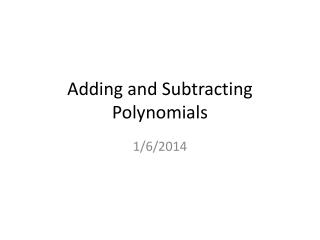DownloadDownload PresentationTélécharger la présentation- - - - - - - - - - - - - - - - - - - - - - - - - - - E N D - - - - - - - - - - - - - - - - - - - - - - - - - - -
Presentation Transcript

1. Example 1 a. Add and – – – 2x3 4x2 x3 6x2 + + + x 9 5x 1 SOLUTION a. – 2x3 4x2 + + x 9 – – x3 6x2 + + 5x 1 – 3x3 2x2 + + 4x 8 Add Polynomials Vertically

2. Example 1 – 5x2 2x 4x2 7x + + 3 – 3 5x2 4x2 2x 7x + + + = – 3 9x2 5x + = Add Polynomials Horizontally b. Add and Group like terms. Combine like terms.

3. Example 2 SOLUTION Align like terms, then add the opposite of the subtracted polynomial. – – 6x3 x2 + 7x 12 – – – 3x3 4x2 + 9x – – – 3x3 5x2 2x 12 Subtract Polynomials (3

4. Example 3 – – 8x2 4x 2x2 6x + 20 + + 14 Use distributive property. = – – 6x 20 14 + + + Group like terms. 8x2 2x2 4x = + + Combine like terms. 10x2 2x 6 = Use the Distributive Property Simplify the expression. a. ( ) ( ) – – 2x2 x x2 3x + + + 4 5 2 7

5. Example 3 – – + – Group like terms. x4 x3 x3 x2 x2 x x 1 + + + = – Combine like terms. x4 2x 1 + = Use the Distributive Property Use distributive property. – – – x4 x3 x2 x x3 x2 x 1 + + + + =